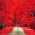how to pass one variable to other page or same page | JS | HTML

How to pass one variable to another page or same page?

﻿
So, Here I have given two file's code where one is data sending file. And another one is data receiving file if we transfer to another page.

index.html

```<form action="#tp" method="get">
<input type="text" name="serialNumber" Value="rajnish" />
<input type="submit" value="Submit" />
</form>

<div id="tp">
<div id="data">
</div>
</div>

<script>
function processForm()
{
var parameters = location.search.substring(1).split("&");
var temp = parameters.split("=");
l = unscape(temp);
document.getElementById("data").innerHTML = l;
}
processForm();
</script>```
Here above code will be used only when you want to pass a variable on one page only.
```<form action="#tp" method="get">
<input type="text" name="serialNumber" Value="rajnish" />
<input type="submit" value="Submit" />
</form>```
Here, I have made one Form Tag for an get user's value in the input box. And for that, i have given form action to "tp" which is id name where the inputted data will show. And for that, we have to give Get/Post method to the form.
```<div id="tp">
<div id="data">
</div>
</div>```
And this the division where the data will print.
```<script>
function processForm()
{
var parameters = location.search.substring(1).split("&");
var temp = parameters.split("=");
l = unscape(temp);
document.getElementById("data").innerHTML = l;
}
processForm();
</script>```
This script tag will fetch that information from URL. after function starts the 'parameters' variable store the search location value with the 'split("&")', Which is used for split the sentence with '&' symbol.
And next 'temp' variable will store the '0th parameters' with an 'split("=")'. and the 'l' will take the 1st value of 'temp' variable and give to 'data' id's element.
so, this was the code of one-page data transferring. But if you want to pass that data to another page then index page will be like:
index.html
```<form action="action.html" method="get">
<input type="text" name="serialNumber" Value="rajnish" />
<input type="submit" value="Submit" />
</form>```
so, here we have to just change the action's value to the page name.
and then action.html will contain rest of the code.
action.html
```<div id="tp">
<div id="data">
</div>
</div>

<script>
function processForm()
{
var parameters = location.search.substring(1).split("&");
var temp = parameters.split("=");
l = unscape(temp);
document.getElementById("data").innerHTML = l;
}
processForm();
</script>```
So, here everything will be same as above. And you can now fetch that data to this page.
You can find this video tutorial of this as well by following:
If you have any questions and queries, comment us below.

1.Hey,
So we are splitting the url by the '&' sign first but we wont have the '&' sign in our url as we are only passing the one value. Then will the code still work??

How to make attractive animation with css

How to make an attractive animation with CSS?

So, here I have attached the code which we'll use in this tutorial. CSS Code:   @keyframes shake {   10%, 90% {     transform: translate3d(-1px, 0, 0);   }
20%, 80% {     transform: translate3d(2px, 0, 0);   }
30%, 50%, 70% {     transform: translate3d(-4px, 0, 0);   }
40%, 60% {     transform: translate3d(4px, 0, 0);   }   }
@keyframes bounce {   10%, 90% {     transform: translate3d(0, 5px, 0);   }
20%, 80% {     transform: translate3d(0, 3px, 0);   }
30%, 50%, 70% {     transform: translate3d(0, 7px, 0);   }
40%, 60% {     transform: translate3d(0, 2px, 0);   }   }
@keyframes circleanimation {     0%{       transform: scale(0.5);       opacity: 0;     }     60%{       transform: scale(1.4);       opacity: 1;     }     100%{       transform: scale(1);     }   }
@keyframes arrow {     0% { border-left: 4.5vw solid #0C6095;}     50% {border-left: 5.5vw solid #0C6095;}     100% {border-left: 4.5vw solid #0C6095;}   }
@keyframes arrow2 {     0% { border-left: 4.5vw …

How to Writing into CSV File with Python Programming

how to write into a CSV file with a Python Programming?

So, Here I have given Python Code and CSV file which I was used in Tutorial. Python Code:import csv with open("data.txt", "r") as dataFile: dataFileReader = csv.reader(dataFile) dataList = [] for row in dataFileReader: if len (row) != 0: dataList = dataList + [row] dataFile.close() print(dataList)name = input("Enter name: ")secondName = input("Enter secondName: ") value1 = input("value1: ") value2 = input("value2: ") value3 = input("value3: ") with open("data.txt", "a") as dataFile: dataFileWriter = csv.writer(dataFile) dataFileWriter.writerow([name, secondName, value1, value2, value3]) dataFile.close()CSV File :ABC, XYZ, 1, 2, 3 PQR, WXY, 4, 5, 6 DEF, JKL, 7, 8, 9This Python code is made for a view, imports, and write the comma separated files in python app. Also with this code user can read that file in the app also…